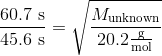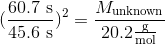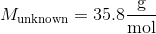# College Chemistry : Effusion

## Example Questions

### Example Question #1 : Effusion

Per Graham's law of effusion, how does the molar mass relate to both the rate and time of effusion?

The molar mass relates inversely to both the rate and time of effusion

The molar mass relates inversely to the rate of effusion and directly to the time of effusion

The molar mass relates directly to both the rate and time of effusion

The molar mass relates directly to the rate of effusion and inversely to the time of effusion

None of these

The molar mass relates inversely to the rate of effusion and directly to the time of effusion

Explanation: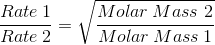This equation explicitly shows how the rate of effusion is inversely proportional to the molar mass of a gas in a gaseous solution.

Because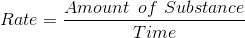, time relates to molar mass by: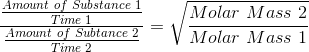Simplifying this equation, we see that: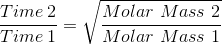As a result, time relates directly to molar mass

### Example Question #2 : Effusion

At the same temperature, an unknown gas effuses at a rate that is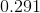times that of oxygen. Find the molar mass, in grams per mole, of the unknown gas.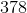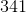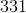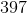Explanation:

Recall Graham's Law of Effusion for two gases, A and B: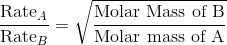From the equation, we know the following: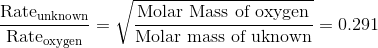Thus, we can solve for the molar mass of the unknown gas. Letbe the molar mass of the unknown gas.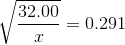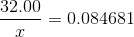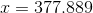Make sure that your answer hassignificant figures.

### Example Question #3 : Effusion

Which of the following is a true statement with regards to the relative effusion rates of oxygen and carbon dioxide?

Cannot be determined from the given information.

Carbon dioxide will effuse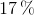faster than oxygen

Oxygen will effuse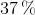faster than carbon dioxide

Carbon dioxide will effusefaster than oxygen

Oxygen will effusefaster than carbon dioxide

Oxygen will effusefaster than carbon dioxide

Explanation:

We're being asked to compare the effusion rates of oxygen and carbon dioxide.

Remember that effusion is the spontaneous movement of a gas through a small hole from one area to another. It's worth noting that at a given temperature, the average speed of all gas molecules in a system is used to calculate the average kinetic energy of the gas particles. This dependence of kinetic energy on temperature means that at a given temperature, any gas particle will have the same kinetic energy.

In this case, we can say that the kinetic energy of oxygen molecules in one system is equal to the kinetic energy of carbon dioxide molecules in another system. Furthermore, since mass is inversely proportional to velocity, identical kinetic energies would mean that as the mass of the gas particles in a system decreases, their velocity (and thus, effusion rates) would increase.

We can use this information to solve for the relative effusion rates between oxygen and carbon dioxide. By setting their kinetic energies equal to each other, we can derive an expression that relates their relative speeds to their relative masses.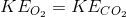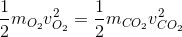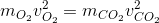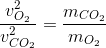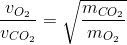Generally speaking, this expression shows how the velocity of any two gasses depends on their mass. In this case, the gasses are oxygen and carbon dioxide.

We can use the periodic table of the elements to find out the mass of each gas, and use that information to calculate the relative effusion rates.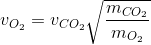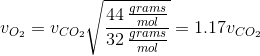This shows that oxygen will effuse at a rate that is aboutfaster than carbon dioxide.

### Example Question #61 : Solutions, States Of Matter, And Thermochemistry

Suppose that gas A effuses at a rate that is twice that of gas B. If the mass of gas A is halved and the mass of gas B is doubled, which of the following correctly describes the new relative effusion rates of these two gasses?

Gas B will now effuse at a ratetimes that of gas A

Gas A will now effuse at a ratetimes that of gas B

Gas B will now effuse at a ratetimes that of gas A

Gas A will now effuse at a ratetimes that of gas B

Gas A will now effuse at a ratetimes that of gas B

Explanation:

For this question, we're given the relative effusion rates for two gasses. We're then told how the mass of each of these gasses is changed, and then we're asked to determine the new relative effusion rates of the two gasses.

First, we can recall the expression that describes the dependence of the effusion rates of two gasses on their mass. Since we're told that the rate of gas A is twice that of gas B, we can write the following expression.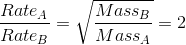Furthermore, since we're told that the mass of gas B is doubled and the mass of gas A is halved, we can determine how the rate will change.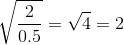Thus, we can see that the rate will change by a factor of two. Hence, the new rate will be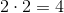. Thus, gas A will now effuse at a ratetimes that of gas B.

### Example Question #62 : Solutions, States Of Matter, And Thermochemistry

A sample of Ne(g) effusses through a tiny hole in 60.7 s. An unknown gas, under identical conditions, effusses in 45.6 s.

What is the molar mass of the unknown gas?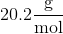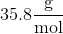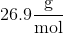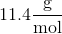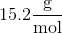Explanation:

To solve this problem use Graham's Law of Effusion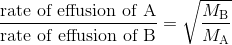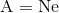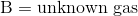By plugging in the values we can rewrite the equation as